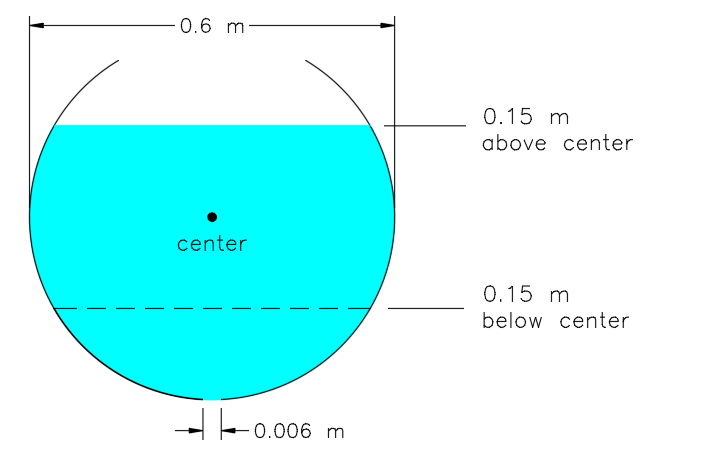# The leaking container

A body of water is contained in a spherical container with diameter 0.6 meter. Suddenly, it gets leaked by a circular hole at the lowest point (the hole is 0.3 meter below center). The hole's diameter is 0.006 meter. The drawing below is not to scale.How long does it take in seconds for water to fall from 0.15 m above center to 0.15 m below center?

Submit the answer as the first 5 significant figures of your result. For example, submit 78940 if you get 78.94067, submit 94084 if you get 940.84058, etc.

Details and assumptions:

Gravitational acceleration $g=9.81\quad m/{ s }^{ 2 }$

The fluid is incompressible, so the volume flow rate is constant at all positions.

Ignore factors such as friction, minor losses, surface tension, etc. so that the flow obeys Bernoulli's equation:

$​{ P }_{ A }+\frac { 1 }{ 2 } { \rho v }_{ A }^{ 2 }+\rho g{ h }_{ A }={ P }_{ B }+\frac { 1 }{ 2 } \rho { v }_{ B }^{ 2 }+\rho g{ h }_{ B }$

Both the water surface and the hole are exposed to open atmosphere.

Though technically, the circular hole will distort the container's shape, making it no longer a sphere and the hole's distance to center is less than 0.3 m. But for simplicity, assume the water inside is always part of a perfect sphere and the hole is exactly 0.3 m below center.

Note:

My solution involves a bit of programming to get through a hard part. If you can solve this manually, please tell me below. Thank you.

×